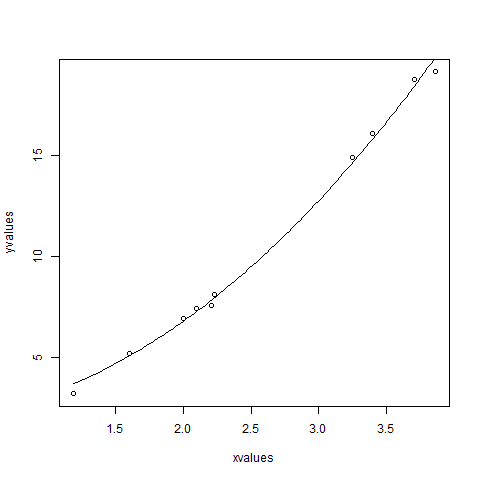# R语言 非线性最小二乘

## 语法

```nls(formula, data, start)
```

• formula是包括变量和参数的非线性模型公式。
• data是用于计算公式中变量的数据框。
• start是起始估计的命名列表或命名数字向量。

## 例

```a = b1*x^2+b2
```

```xvalues <- c(1.6,2.1,2,2.23,3.71,3.25,3.4,3.86,1.19,2.21)
yvalues <- c(5.19,7.43,6.94,8.11,18.75,14.88,16.06,19.12,3.21,7.58)

# Give the chart file a name.
png(file = "nls.png")

# Plot these values.
plot(xvalues,yvalues)

# Take the assumed values and fit into the model.
model <- nls(yvalues ~ b1*xvalues^2+b2,start = list(b1 = 1,b2 = 3))

# Plot the chart with new data by fitting it to a prediction from 100 data points.
new.data <- data.frame(xvalues = seq(min(xvalues),max(xvalues),len = 100))
lines(new.data\$xvalues,predict(model,newdata = new.data))

# Save the file.
dev.off()

# Get the sum of the squared residuals.
print(sum(resid(model)^2))

# Get the confidence intervals on the chosen values of the coefficients.
print(confint(model))
```

``` 1.081935
Waiting for profiling to be done...
2.5%    97.5%
b1 1.137708 1.253135
b2 1.497364 2.496484
```## 【阿里云】双11拼团上云低至85元

#### 1核2G1M40G盘，84元/1年，254元/3年